Editor Ratings:
User Ratings:
[Total: 3 Average: 5]

You can solve mathematical expressions on Facebook Messenger using Calcbot – a simple bot for Facebook Messenger. It can also be used to convert measurement units and currencies, and solve simple trigonometric expressions. It will also assist you in calculating the amount of tip to be paid and splitting up your bills.

This Facebook Messenger Maths bot is quite easy to use as you just have to send your maths expression or conversion expression  as a message to CalcBot, and it will reply with the answer instantly.

Keep reading to know how you can calculate and convert units using this free Facebook messenger bot.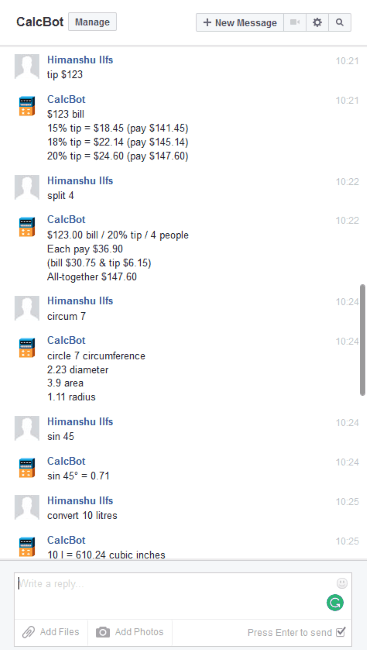This Facebook messenger bot really proves its  worth as you do not have to leave facebook or use another application for your calculation tasks. You can now perform most of your calculations and chat with your friends side by side.

## How to get started with this Facebook messenger bot:

Basically, this Facebook messenger bot is a Facebook page which instantly replies with the answers of your mathematical expressions. You don’t have to like this page for getting your queries solved. You just have to initiate a conversation by sending a message to CalcBot Facebook page. You can send a help message and CalcBot will reply with syntaxes that you have to follow to perform various calculations.

Let’s see how you can solve different types of mathematical expressions using Calc.

• Numeric Expressions: You can calculate simple numeric expressions (like 12+34) and compound numeric expressions (like 34+23/4-14*45) on Facebook Messenger using CalcBot. You just need to send an expression directly as a message to Calcbot and it will instantly reply with the answer.• Trigonometric Expressions: You can solve a trigonometry equation of maximum 2 terms on CalcBot.
• Syntax for 1 term trigonometry equation: “Trigonometry function” (Sin 45): It will display angle value of that trigonometry function.
• Syntax for 2 term trigonometry equation: “Trigonometry function1” “Arithmetic operator” “Trigonometry function2” (cos 45 + cot 90): It will display angle value after performing the arithmetic operation on both trigonometric functions.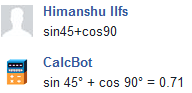• Percentage: To calculate percentage, follow this syntax: “Number%” of Total Number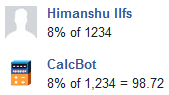• Currency: You can easily convert a currency of one country to other. Follow this syntax “Currency Sign”Amount to Desired currency ( \$100 to euros).• Tips & Bills: To calculate Tips, follow this syntax: “tip” “amount of bill” “percentage”. It will then display the total amount to be paid.
Now if you want to split up your bill, then follow this syntax: “split” “Number of Persons”. It will then display total amount to be paid by each person.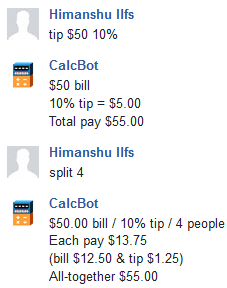• Conversion: To convert a measurement unit to another, follow this syntax: “Convert” Numeric value “SI unit” to “SI unit of desired quantity” (convert 10 mm to km).
To convert a measurement into all other measurement units, follow this syntax: “Convert” Numeric value “SI unit”.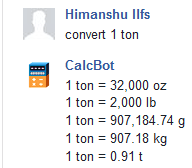• Calculate Diameter, Radius, and Area of Circle by specifying its circumference: Follow this syntax: “circum” “magnitude”.## Verdict:

I really liked this Facebook messenger bot as it automatically converts different measurement units and different currencies by a single command message. It was fun to use and calculate with CalcBot as it is very interactive and it replied answers instantly. But its best feature is that you can perform all the calculations without leaving Facebook or opening any other application. However, it just can be used for simple calculations, but this limitation can be neglected as you can work on Facebook and calculate at the same time.

Start using this Facebook messenger bot from here.